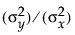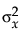Standard least square fitting assumes that the X variable is fixed and the Y variable is a function of X plus error. If there is random variation in the measurement of X, you should fit a line that minimizes the sum of the squared perpendicular differences. See Figure 4.49. However, the perpendicular distance depends on how X and Y are scaled, and the scaling for the perpendicular is reserved as a statistical issue, not a graphical one.
Figure 4.49 Line Perpendicular to the Line of Fit
The fit requires that you specify the ratio of the variance of the error in Y to the error in X. This is the variance of the error, not the variance of the sample points, so you must choose carefully. The ratiois infinite in standard least squares becauseis zero. If you do an orthogonal fit with a large error ratio, the fitted line approaches the standard least squares line of fit. If you specify a ratio of zero, the fit is equivalent to the regression of X on Y, instead of Y on X.
The most common use of this technique is in comparing two measurement systems that both have errors in measuring the same value. Thus, the Y response error and the X measurement error are both the same type of measurement error. Where do you get the measurement error variances? You cannot get them from bivariate data because you cannot tell which measurement system produces what proportion of the error. So, you either must blindly assume some ratio like 1, or you must rely on separate repeated measurements of the same unit by the two measurement systems.
An advantage to this approach is that the computations give you predicted values for both Y and X; the predicted values are the point on the line that is closest to the data point, where closeness is relative to the variance ratio.

Help created on 7/12/2018